## RaysWebClass.Com

### Lesson 6:   HTML Tables (Part One)

1. A Simple Table

 A
The Code is as follows:

<table border ='1'>
<tr>
<td>A</td>
</tr>
</table>
Note that We open the table with the <table> tag. By adding the border ="1" attribute, we can see the border (a border ="0" would turn the table border off and all you would see is the data inside of the table. This is (sometimes) a desirable effect.) Next, we open the table row with the <tr> and then the table data cell <td>. Enter the Data ("A") and close the table data </td> and then the table row </tr> and then the table </table>.
HTML tables have five tags:

• <table> is the opening table tags.

• <tr> starts a table row.

• <td> starts a table data cell.

• <th> starts a table header.

• <caption> is where you place a caption above or below the table.

2. A Normal Table

My Table
Boys Girls
A B
C D
Code:

<table border='1'>
<caption align='bottom'>My Table</caption>
<tr>
<th>Boys</th>
<th>Girls</th>
</tr>
<tr>
<td>A</td>
<td>B</td>
</tr>
<tr>
<td>C</td>
<td>D</td>
</tr>
</table>

3. A Complex Table

Languages English Bagrable* 1 "One" "Bagrable"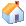"House"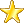"Star"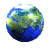"Earth"
In the table below notce the first cell in this complex table is two rows high, we have merged these two cells together. The table attribute to do this is rowspan="2". The second cell on the top row is two columns wide, the table attribute for this is colspan="2". And the lower right cell is four rows high: rowspan="4".
Code:

<table border='1'>
<tr>
<td rowspan='2'>   </td>
<th colspan='2'>Languages</th>
</tr>
<tr>
<th>English</th>
<th>Bagrable<sup>*</sup></th>
</tr>
<tr>
<td align='center'> 1 </td>
<td> "One" </td>
<td rowspan='4'> "Bagrable" </td>
</tr>
<tr>
<td align='center'><img src='tbl1.gif'></td>
<td> "House" </td>
</tr>
<tr>
<td align='center'><img src='tbl2.gif'></td>
<td> "Star" </td>
</tr>
<tr>
<td align='center'><img src='tbl3.gif'></td>
<td> "Earth" </td>
</tr>
</table>

This table is three columns wide and six rows high. Look at the first row in the code. It has only two <td> tags (the second is a <th> but that still count as a tag!) How is that possible? Look more closely at the second tag and you'll see why, the second tag has a colspan="2" that means this second tag spans two columns, thus setting the column count for the first row to three columns wide. See it?
Also notice the first <td> has a rowspan="2". That means this <td> will be the first <td> in the first row as well as the first <td> in the second row. Look now at your second row and you can see there are only two <td> tags there. These are the second and third <td>'s in that row, making the column count for the second row three as well.
The third row in the table has all three <td> tags, but the last <td> has a rowspan="4". That means for this row and the next three rows in this <td> will be the last cell in each row.
The fourth, fifth, and sixth rows have only two <td> tags in each - the first and second cells. The Last cell has already been defined in row three.
Try coding this table: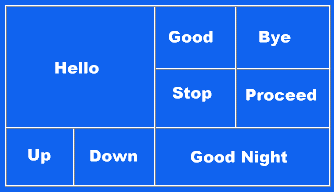## Web Page Design: Exercise 6

Let's add content to the Products page:
Copy this image to the images folder if it is not already there: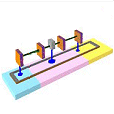NOTE: You may have to remove the  from the name of the copied image.
For example, Doodad.gif may copy over to the images folder as Doodad.gif

Add this content to the Products page between the Main Page Comments:

<tr>
<td colspan='4'><hr></td>
</tr>
<tr>
<td valign='top'>
A Doodad is a long plank with four square blocks on a rod suspended, on
three legs, above the plank.
</td>
<td valign='top' align='right'> <b>\$45.99</b> </td>
</tr>
<tr>
<td colspan='4'><hr></td>
</tr>
<tr>
<td valign='top'>
A Gadget is a round thing with legs connected to two loops on top
of another round cylinder thing.
</td>
<td valign='top' align='right'> <b>\$599.00</b> </td>
</tr>
<tr>
<td colspan='4'><hr></td>
</tr>
<tr>
<td> <img src='images/Widget.gif'> </td>
<td valign='top'> <b>Widget</b> </td>
<td valign='top'>
A Widget is three long slender slats and a shorter fat slat on top of
a pole connected to a rod with a small wheel connected to a large wheel
and axle.
</td>
<td valign='top' align='right'> <b>\$399.00</b> </td>
</tr>
<tr>
<td colspan='4'>
<hr>
<small>
<a href='http://demonstrations.wolfram.com/TheStraightLineAsARoulette/'>
Wolfram Images</a>
</small>
</td>
</tr>
</table>